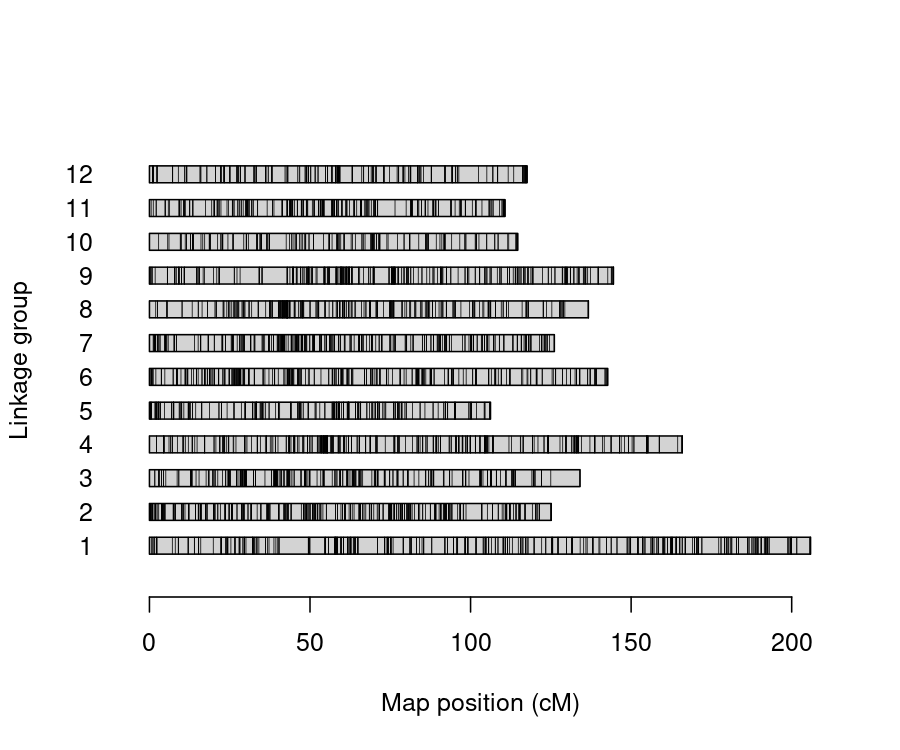# Introduction

The R package qtlpoly (v. 0.2.4) (Pereira et al. 2020) is an under development software to map multiple quantitative trait loci (QTL) in full-sib families of outcrossing autopolyploid species. It is based on the following random-effect model:

$\boldsymbol{y} = \boldsymbol{1}\mu + \sum_{q=1}^Q \boldsymbol{g}_q + \boldsymbol{e}$

where the vector of phenotypic values from a specific trait $$\boldsymbol{y}$$ is a function of the fixed intercept $$\mu$$, the $$q = 1, \dots, Q$$ random QTL effects $$\boldsymbol{g}_q \sim \mathcal{N}(\boldsymbol{0}, \boldsymbol{G}_q\sigma^2_q)$$, and the random environmental error $$\boldsymbol{e} \sim \mathcal{N}(\boldsymbol{0}, \boldsymbol{I}\sigma^2)$$. $$\boldsymbol{G}_q$$ is the additive relationship matrix between full-sibs derived from the genotype conditional probabilities of QTL $$q$$. See model details in Pereira et al. (2020).

Variance components associated with putative QTL ($$\sigma^2_q$$) are tested using score statistics, as proposed in Qu, Guennel, and Marshall (2013). Final models are fitted using residual maximum likelihood (REML) from the R package sommer (v. 4.1) (Covarrubias-Pazaran 2016). Plots for visualizing the results are based on ggplot2 (v. 3.1.0) (Wickham 2016).

This tutorial used R version 4.2.2 Patched (2022-11-10 r83330) running on Ubuntu 20.04 LTS (64-bit).

## Install and load the qtlpoly package and data

qtlpoly package is available in its stable version on CRAN and an under-development version at GitHub. You can install the stable version from CRAN by running:

> install.packages("qtlpoly")

To use the development version, please run the following commands to install qtlpoly and all of its dependencies:

> ## install.packages('devtools')
> devtools::install_github("gabrielgesteira/qtlpoly")

Then, use the function library() – or require() – to load the package:

> library(qtlpoly)

qtlpoly includes preloaded datasets that are simpler, so one can run the functions along with this tutorial using a regular personal computer (with 4 cores and 6 GB of RAM, minimum). For real data analyses, one may need to run an R script in a cluster with more cores and RAM. In general, the computational needs depend on ploidy level, population size, and the number of markers.

The dataset used in this tutorial was based on a full-sib family ($$N = 156$$) from a biparental potato population. You can view the maps with mappoly after installing it:

> ## install.packages('mappoly')
> library(mappoly)
## Warning in fun(libname, pkgname): couldn't connect to display ":0"
## =====================================================
## MAPpoly Package [Version 0.3.22022-12-02 08:00:02 UTC]
> plot_map_list(maps4x)> plot(maps4x[])